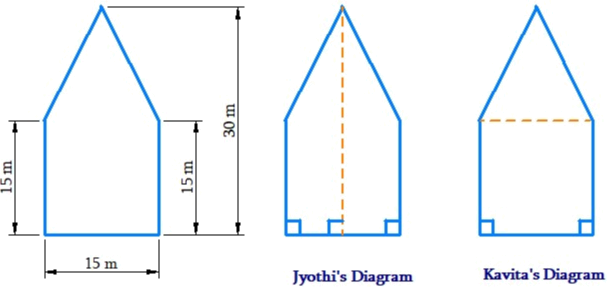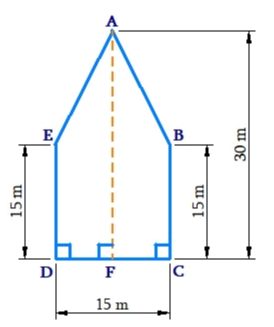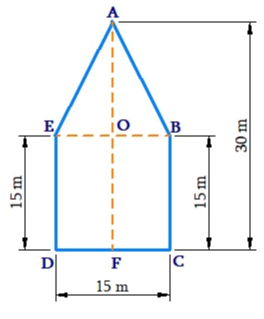# Ex.11.2 Q10 Mensuration Solution - NCERT Maths Class 8

Go back to  'Ex.11.2'

## Question

There is a pentagonal shaped park as shown in the figure. For finding its area Jyoti and Kavita divided it in two different ways. Find the area of this park using both ways. Can you suggest some other way of finding its area?

Video Solution
Mensuration
Ex 11.2 | Question 10

## Text Solution

What is Known?

The park is pentagonal shape and dimension of the park is given.

What is unknown?

Area of the park

Reasoning:

Visually the pentagon is divided into two equal trapeziums or into one triangle and one square. So, the area of the pentagon will be sum of the area of triangle and area of the square.

Steps:(i) Jyoti’s way of triangle area is as follows

Area of pentagon $$ABCDE\,=$$ Area of trapezium $$ABCD\,+$$ Area of trapezium $$AEDF$$\begin{align}& = \begin{bmatrix} \frac{1}{2} \times (AF + BC) \times FC +\\\frac{1}{2} \times (AF \times BC) \times DF\end{bmatrix}\\& =\begin{bmatrix} \frac{1}{2} \times (13\,{\rm{m}} + 15\,{\rm{m}}) \times \frac{{15}}{2}\,{\rm{m}} +\\ \frac{1}{2} \times (30 + 15{\rm{m}}) \times \frac{{15}}{2}{\rm{m}}\end{bmatrix}\\& = 2 \times \frac{1}{2}(30\,{\rm{m}} + 15\,{\rm{m}}) \times \frac{{15}}{2}\\& = 45\,{\rm{m}} \times 7.5\,{\rm{m}}\\& = 337.5\,{{\rm{m}}^2}\end{align}

Thus, area of the pentagonal shaped park according to Jyoti’s way is $$337.5\,\rm{m^2}$$

(ii) Kavitha’s way of finding area is as follows.

Area of pentagon $$ABCDE\;=$$ Area of triangle $$ABE\; +$$ Area of square $$EBDC$$

\begin{align}& = \! \frac{1}{2} \! \times \! BE \! \times \! (AF \! - \! OF) \! + \! FC \! \times \! BC\\ &= \! \frac{1}{2} \! \times \! 15 \! \times \! (30 \! - \! 15) \! + \! (15 \! \times \! 15\,\,{\rm{m}})\\ &= \! \left( {\frac{1}{2} \! \times \! 15 \! \times \! 15} \right){{\rm{m}}^2} \! + \! 225\,\,{{\rm{m}}^2}\\ &= \! 112.5\,\,{{\rm{m}}^2} \! + \! 225\,\,{{\rm{m}}^2}\\& = \! 337.5\,\,{{\rm{m}}^2}\end{align}

Thus, the area of the pentagonal shaped park according to Kavitha’s way is $$337.5 \,\rm{m^2}$$

Another way of finding its area is follows.Area of pentagon $$ABCDE \;=$$Area of rectangle $$GFCD – 2$$(Area of $$\Delta AGE$$)

\begin{align} &= \! 30\,{\rm{m}} \! \times \! 15\,\,{\rm{m}} \! - \! 2 \! \times \! \begin{bmatrix} {\frac{1}{2} \! \times \! 7.5 \! \times \! 1.5} \end{bmatrix}\\& = \! 450\,{{\rm{m}}^2} \! + \! 112.5\,\,{{\rm{m}}^2}\\& = \! 337.5\,{\rm{c}}{{\rm{m}}^2}\end{align}

Learn from the best math teachers and top your exams

• Live one on one classroom and doubt clearing
• Practice worksheets in and after class for conceptual clarity
• Personalized curriculum to keep up with school